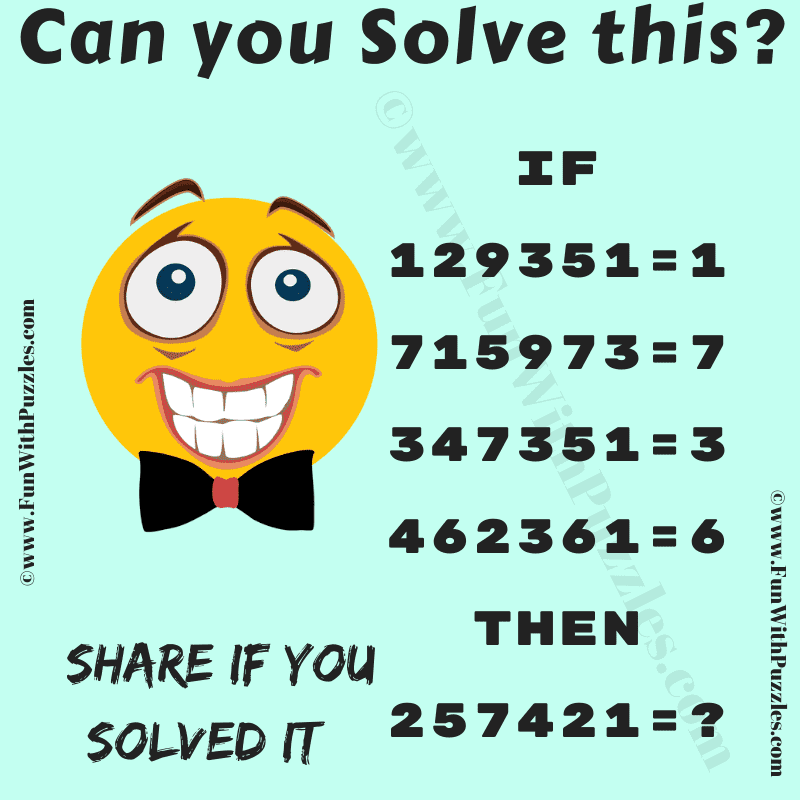This is the fun logic puzzle of mathematics that will challenge your brain. This maths logic puzzle is for school-going students. Solving these kinds of logical reasoning and mathematical puzzles will help you to improve your IQ. Let's see if you can find the hidden pattern in the given number equations. Once you are able to crack the logical reasoning of these number equations, find the value of the missing number that will replace the question mark.Can you find the Missing Number?

The answer to this "Fun Logic Puzzle of Math", can be viewed by clicking on the answer button.